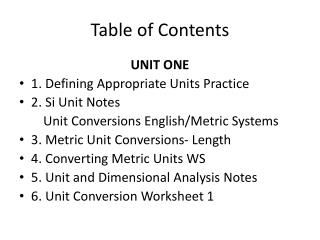DownloadDownload PresentationDownload Presentation- - - - - - - - - - - - - - - - - - - - - - - - - - - E N D - - - - - - - - - - - - - - - - - - - - - - - - - - -
##### Presentation Transcript

1. Table of Contents UNIT ONE • 1. Defining Appropriate Units Practice • 2. Si Unit Notes Unit Conversions English/Metric Systems • 3. Metric Unit Conversions- Length • 4. Converting Metric Units WS • 5. Unit and Dimensional Analysis Notes • 6. Unit Conversion Worksheet 1

2. 7. Dimensional Analysis Worksheet • 8. Unit Conversion Practice #2 • 9. Unit Conversions Quizee • 10. Interpreting Language in Math Expressions Notes • 11. Identifying Parts of an Algebraic Expression WS • 12. Identifying Parts and Translating Expressions WS • 13. Algebra Review

3. 14. How do you solve multi step linear equations notes? • 15. Solving equations review worksheet • 16. Solving Multi – Step Equations worksheet • 17. Inequalities Practice • 18. Inequalities • 19. Multi – Step Inequalities • 20. Literal Equations Notes • 21. Equations and Inequalities Quiz • 22. Literal Equation Practice • 23. Solving for the Missing Variable Word Problems

4. 24. Extra Order of Operations and Solving Equations Practice • 25. Creating Equations Notes • 26. Translate and Solve Extra Practice WS • 27. Linear Equations and Inequalities WS • 28. Packet 22: Inequality Word Problems • 29. Inequality Word Problem Homework • 30. Lucy’s Linear Equations and Inequalities • 31. Creating Equations Practice WS • 32. Writing Equations and Inequalities HW

5. 33. Unit Conversions/ Literal Eq. / Creating Equations Quiz • 34. Inequalities Word Problems • 35. Properties of Exponents #1 • 36. Properties of Exponents #2 • 37. Properties of Exponents HW • 38. Solving Exponential Equations Notes and Chart • 39. Solving Exponential Equations Practice • 40. Solving Exponential Equations • 41. Solving Exponential Functions HOMEWORK

6. 42. YoYo and Paper Task • 43. Exponential Application Notes • 44. Solving Exponential Equations Quiz • 45. Exponential Growth and Decay WS • 46. Exponential Growth and Decay (8.8) • 47. Graphing Lines in Slope Intercept Form Notes • 48.Graphing Lines • 49. Graphing Exponential Functions GO • 50. Graphing Exponential Functions Notes • 51. Unit 1 Quiz Homework

7. 52. Unit 1 Assessment Packet 1 • 53. Unit 1 Assessment Packet 2 • 54. Unit 1 Study Guide • 55. Unit 1 Test Review # 2

8. Unit 2 Reasoning with Equations and Inequalities • 1. Fall Break Packet • 2. Word Scramble • 3. Hoo’s There? • 4. Solving Equations with Justification Notes • 5. Properties of Equality Homework • 6. Algebraic Properties Worksheet • 7. Solve for Y and Graph • 8. Graphing Lines Packet

9. 9. Introduction to Systems of Linear Equations Notes • 10. Solving Systems by Graphing Notes • 11. Solving Systems by Graphing Homework • 12. Systems of Equations TARGET PRACTICE • 13. Systems of Equations Graphing Quizzee • 14. What Were the Headlines…? Worksheet • 15. Solving Systems by Substitution Notes • 16. Solving Systems of Equations by Substitution Worksheet • 17. QR Code Substitution Activity

10. 18. pg. 144 8-16 Even homework • 19. Substitution Quizzee • 20. Elimination Notes • 21. Solving Systems by Elimination WS • 22. pg. 151 #1-19 Odd homework • 23. Math Lib • 24. Elimination Homework • 25. Solving Systems Quiz • 26. Notes for Graphing Linear Inequalities • 27. Graphing Inequalities Review

11. 28. Graphing System of Inequalities Notes • 29. Systems of Inequalities Worksheet • 30. System of Inequalities Scavenger Hunt • 31. Color By Numbers • 32. Writing System of Equations Notes • 33. Solving Systems of Equations WS • 34. System of Equations Word Problems • 35. Solving System Word Problem Packet • 36. Writing Systems from Word Problem Practice Worksheet

12. 37. Unit 2 Study Guide • 38. Unit 5 Test Practice • 39. Reasoning with Equations and Inequalities Practice – Homework • 40. Unit 2 Topics of Study • 41. Systems of Equations Road Map • 42. Solving Systems Hang Man – Extra Credit • 43. Unit 2 Test